# 动画+原理+代码，解读十大经典排序算法

+关注继续查看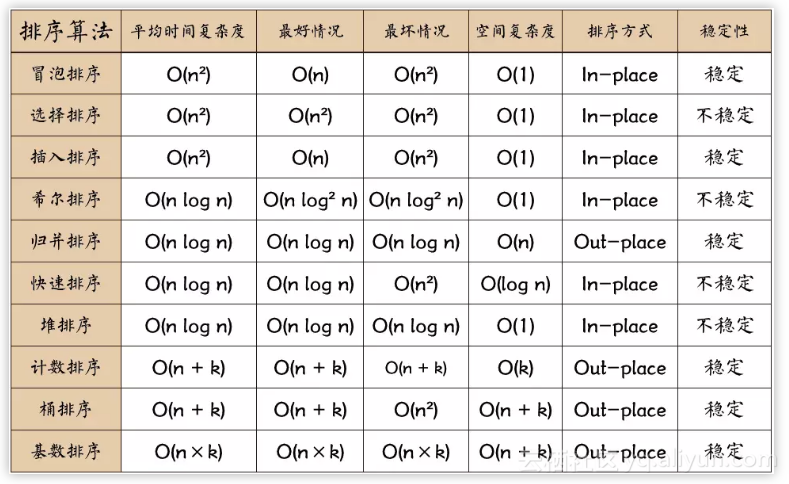●  平方阶 (O(n2)) 排序 各类简单排序：直接插入、直接选择和冒泡排序。
●  线性对数阶 (O(nlog2n)) 排序 快速排序、堆排序和归并排序。
●  O(n1+§)) 排序，§ 是介于 0 和 1 之间的常数。 希尔排序。
●  线性阶 (O(n)) 排序 基数排序，此外还有桶、箱排序。

n：数据规模

k：“桶”的个数

In-place：占用常数内存，不占用额外内存

Out-place：占用额外内存

1. 算法步骤

●  比较相邻的元素。如果第一个比第二个大，就交换他们两个。
●  对每一对相邻元素作同样的工作，从开始第一对到结尾的最后一对。这步做完后，最后的元素会是最大的数。
●  针对所有的元素重复以上的步骤，除了最后一个。
●  持续每次对越来越少的元素重复上面的步骤，直到没有任何一对数字需要比较。

2. 动图演示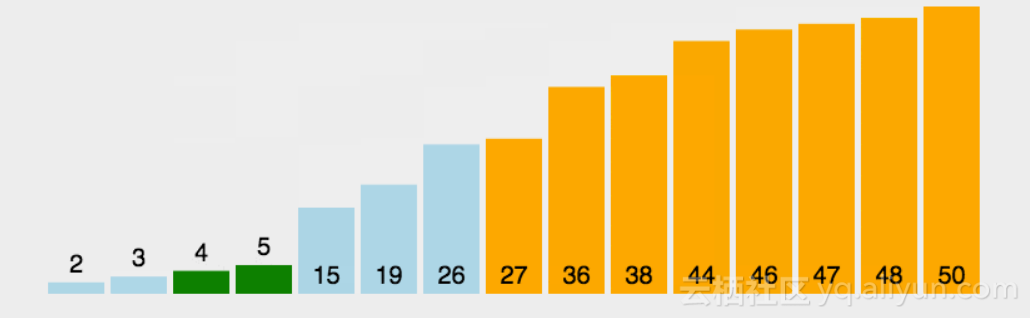3. 什么时候最快

4. 什么时候最慢

5. Java 代码实现

public class BubbleSort implements IArraySort {

@Override
public int[] sort(int[] sourceArray) throws Exception {
// 对 arr 进行拷贝，不改变参数内容
int[] arr = Arrays.copyOf(sourceArray, sourceArray.length);

for (int i = 1; i < arr.length; i++) {
// 设定一个标记，若为true，则表示此次循环没有进行交换，也就是待排序列已经有序，排序已经完成。
boolean flag = true;

for (int j = 0; j < arr.length - i; j++) {
if (arr[j] > arr[j + 1]) {
int tmp = arr[j];
arr[j] = arr[j + 1];
arr[j + 1] = tmp;

flag = false;
}
}

if (flag) {
break;
}
}
return arr;
}
}

1. 算法步骤

●  首先在未排序序列中找到最小（大）元素，存放到排序序列的起始位置
●  再从剩余未排序元素中继续寻找最小（大）元素，然后放到已排序序列的末尾。
●  重复第二步，直到所有元素均排序完毕。

2. 动图演示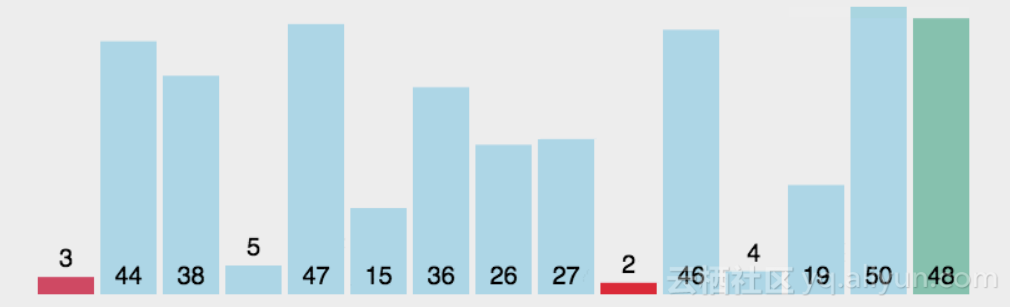3. Java 代码实现

public class SelectionSort implements IArraySort {

@Override
public int[] sort(int[] sourceArray) throws Exception {
int[] arr = Arrays.copyOf(sourceArray, sourceArray.length);

// 总共要经过 N-1 轮比较
for (int i = 0; i < arr.length - 1; i++) {
int min = i;

// 每轮需要比较的次数 N-i
for (int j = i + 1; j < arr.length; j++) {
if (arr[j] < arr[min]) {
// 记录目前能找到的最小值元素的下标
min = j;
}
}

// 将找到的最小值和i位置所在的值进行交换
if (i != min) {
int tmp = arr[i];
arr[i] = arr[min];
arr[min] = tmp;
}

}
return arr;
}
}

1. 算法步骤

●  将第一待排序序列第一个元素看做一个有序序列，把第二个元素到最后一个元素当成是未排序序列。
●  从头到尾依次扫描未排序序列，将扫描到的每个元素插入有序序列的适当位置。（如果待插入的元素与有序序列中的某个元素相等，则将待插入元素插入到相等元素的后面。）

2. 动图演示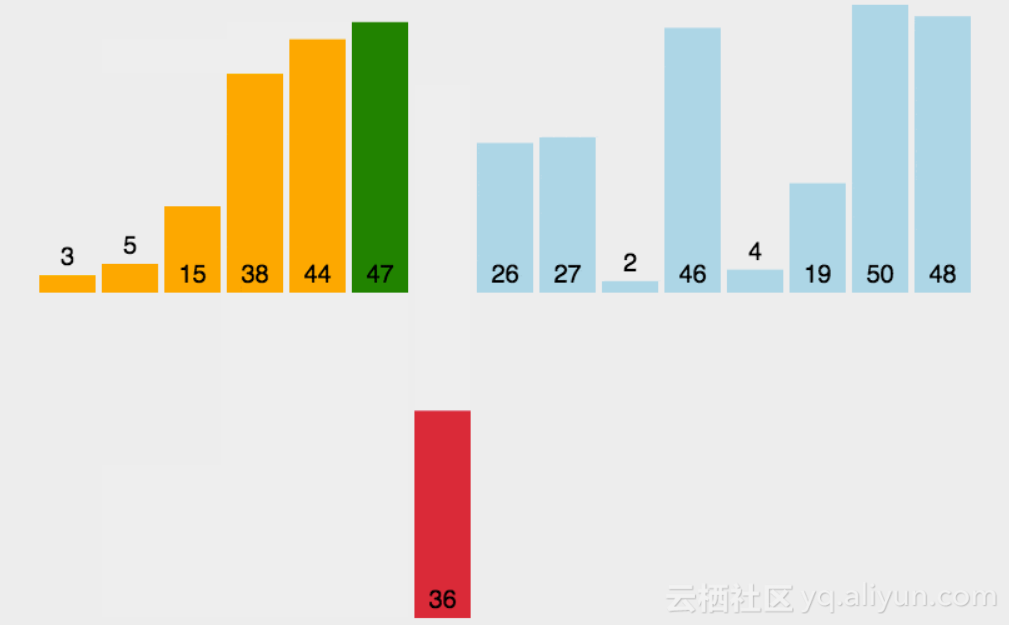3. Java 代码实现

public class InsertSort implements IArraySort {

@Override
public int[] sort(int[] sourceArray) throws Exception {
// 对 arr 进行拷贝，不改变参数内容
int[] arr = Arrays.copyOf(sourceArray, sourceArray.length);

// 从下标为1的元素开始选择合适的位置插入，因为下标为0的只有一个元素，默认是有序的
for (int i = 1; i < arr.length; i++) {

// 记录要插入的数据
int tmp = arr[i];

// 从已经排序的序列最右边的开始比较，找到比其小的数
int j = i;
while (j > 0 && tmp < arr[j - 1]) {
arr[j] = arr[j - 1];
j--;
}

// 存在比其小的数，插入
if (j != i) {
arr[j] = tmp;
}

}
return arr;
}
}

●  插入排序在对几乎已经排好序的数据操作时，效率高，即可以达到线性排序的效率；
●  但插入排序一般来说是低效的，因为插入排序每次只能将数据移动一位；

1. 算法步骤

●  选择一个增量序列 t1，t2，……，tk，其中 ti > tj, tk = 1；
●  按增量序列个数 k，对序列进行 k 趟排序；
●  每趟排序，根据对应的增量 ti，将待排序列分割成若干长度为 m 的子序列，分别对各子表进行直接插入排序。仅增量因子为 1 时，整个序列作为一个表来处理，表长度即为整个序列的长度。

2. Java 代码实现

public class ShellSort implements IArraySort {

@Override
public int[] sort(int[] sourceArray) throws Exception {
// 对 arr 进行拷贝，不改变参数内容
int[] arr = Arrays.copyOf(sourceArray, sourceArray.length);

int gap = 1;
while (gap < arr.length) {
gap = gap * 3 + 1;
}

while (gap > 0) {
for (int i = gap; i < arr.length; i++) {
int tmp = arr[i];
int j = i - gap;
while (j >= 0 && arr[j] > tmp) {
arr[j + gap] = arr[j];
j -= gap;
}
arr[j + gap] = tmp;
}
gap = (int) Math.floor(gap / 3);
}

return arr;
}
}

●  自上而下的递归（所有递归的方法都可以用迭代重写，所以就有了第 2 种方法）；
●  自下而上的迭代；

However, it is not possible to do so in JavaScript, as the recursion goes too deep for the language to handle.

1. 算法步骤

●  申请空间，使其大小为两个已经排序序列之和，该空间用来存放合并后的序列；
●  设定两个指针，最初位置分别为两个已经排序序列的起始位置；
●  比较两个指针所指向的元素，选择相对小的元素放入到合并空间，并移动指针到下一位置；
●  重复步骤 3 直到某一指针达到序列尾；
●  将另一序列剩下的所有元素直接复制到合并序列尾。

2. 动图演示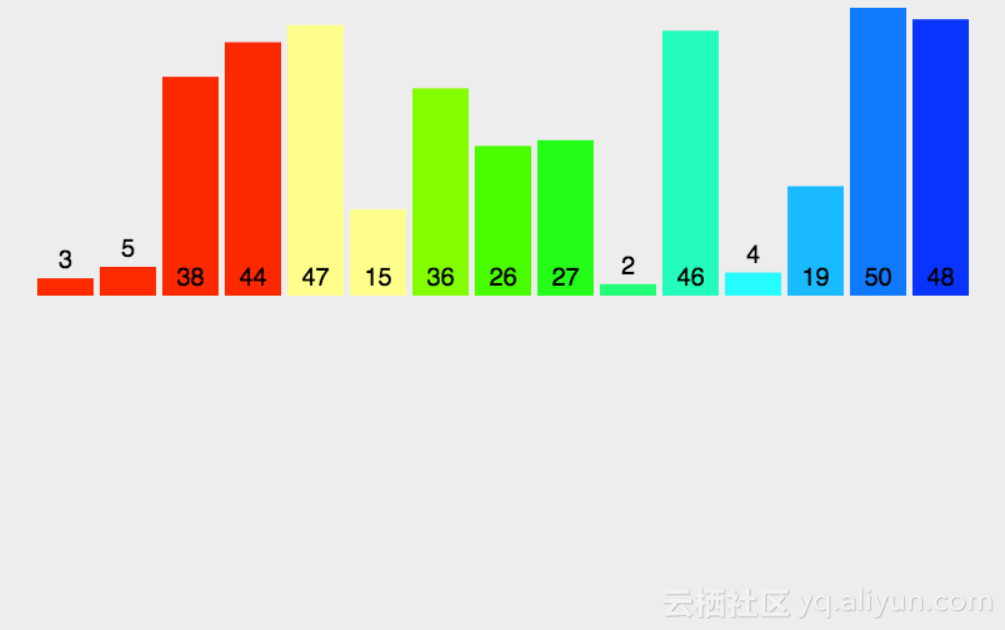3. Java 代码实现

public class MergeSort implements IArraySort {

@Override
public int[] sort(int[] sourceArray) throws Exception {
// 对 arr 进行拷贝，不改变参数内容
int[] arr = Arrays.copyOf(sourceArray, sourceArray.length);

if (arr.length < 2) {
return arr;
}
int middle = (int) Math.floor(arr.length / 2);

int[] left = Arrays.copyOfRange(arr, 0, middle);
int[] right = Arrays.copyOfRange(arr, middle, arr.length);

return merge(sort(left), sort(right));
}

protected int[] merge(int[] left, int[] right) {
int[] result = new int[left.length + right.length];
int i = 0;
while (left.length > 0 && right.length > 0) {
if (left <= right) {
result[i++] = left;
left = Arrays.copyOfRange(left, 1, left.length);
} else {
result[i++] = right;
right = Arrays.copyOfRange(right, 1, right.length);
}
}

while (left.length > 0) {
result[i++] = left;
left = Arrays.copyOfRange(left, 1, left.length);
}

while (right.length > 0) {
result[i++] = right;
right = Arrays.copyOfRange(right, 1, right.length);
}

return result;
}

}

1. 算法步骤

●  从数列中挑出一个元素，称为 “基准”（pivot）;
●  重新排序数列，所有元素比基准值小的摆放在基准前面，所有元素比基准值大的摆在基准的后面（相同的数可以到任一边）。在这个分区退出之后，该基准就处于数列的中间位置。这个称为分区（partition）操作；
●  递归地（recursive）把小于基准值元素的子数列和大于基准值元素的子数列排序；

2. 动图演示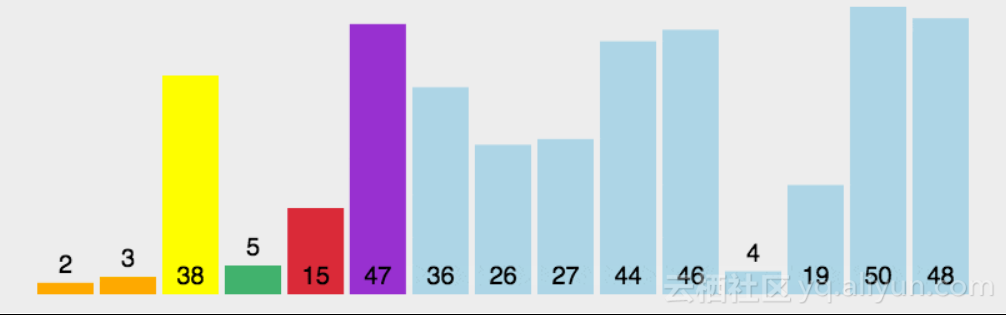3. Java 代码实现

public class QuickSort implements IArraySort {

@Override
public int[] sort(int[] sourceArray) throws Exception {
// 对 arr 进行拷贝，不改变参数内容
int[] arr = Arrays.copyOf(sourceArray, sourceArray.length);

return quickSort(arr, 0, arr.length - 1);
}

private int[] quickSort(int[] arr, int left, int right) {
if (left < right) {
int partitionIndex = partition(arr, left, right);
quickSort(arr, left, partitionIndex - 1);
quickSort(arr, partitionIndex + 1, right);
}
return arr;
}

private int partition(int[] arr, int left, int right) {
// 设定基准值（pivot）
int pivot = left;
int index = pivot + 1;
for (int i = index; i <= right; i++) {
if (arr[i] < arr[pivot]) {
swap(arr, i, index);
index++;
}
}
swap(arr, pivot, index - 1);
return index - 1;
}

private void swap(int[] arr, int i, int j) {
int temp = arr[i];
arr[i] = arr[j];
arr[j] = temp;
}

}

●  大顶堆：每个节点的值都大于或等于其子节点的值，在堆排序算法中用于升序排列；
●  小顶堆：每个节点的值都小于或等于其子节点的值，在堆排序算法中用于降序排列；

1. 算法步骤

●  创建一个堆 H[0……n-1]；
●  把堆首（最大值）和堆尾互换；
●  把堆的尺寸缩小 1，并调用 shift_down(0)，目的是把新的数组顶端数据调整到相应位置；
●  重复步骤 2，直到堆的尺寸为 1。

2. 动图演示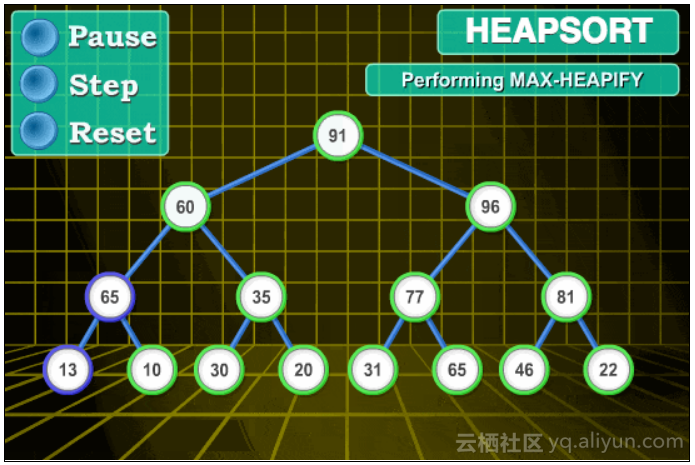3. Java 代码实现

public class HeapSort implements IArraySort {

@Override
public int[] sort(int[] sourceArray) throws Exception {
// 对 arr 进行拷贝，不改变参数内容
int[] arr = Arrays.copyOf(sourceArray, sourceArray.length);

int len = arr.length;

buildMaxHeap(arr, len);

for (int i = len - 1; i > 0; i--) {
swap(arr, 0, i);
len--;
heapify(arr, 0, len);
}
return arr;
}

private void buildMaxHeap(int[] arr, int len) {
for (int i = (int) Math.floor(len / 2); i >= 0; i--) {
heapify(arr, i, len);
}
}

private void heapify(int[] arr, int i, int len) {
int left = 2 * i + 1;
int right = 2 * i + 2;
int largest = i;

if (left < len && arr[left] > arr[largest]) {
largest = left;
}

if (right < len && arr[right] > arr[largest]) {
largest = right;
}

if (largest != i) {
swap(arr, i, largest);
heapify(arr, largest, len);
}
}

private void swap(int[] arr, int i, int j) {
int temp = arr[i];
arr[i] = arr[j];
arr[j] = temp;
}

}

1. 动图演示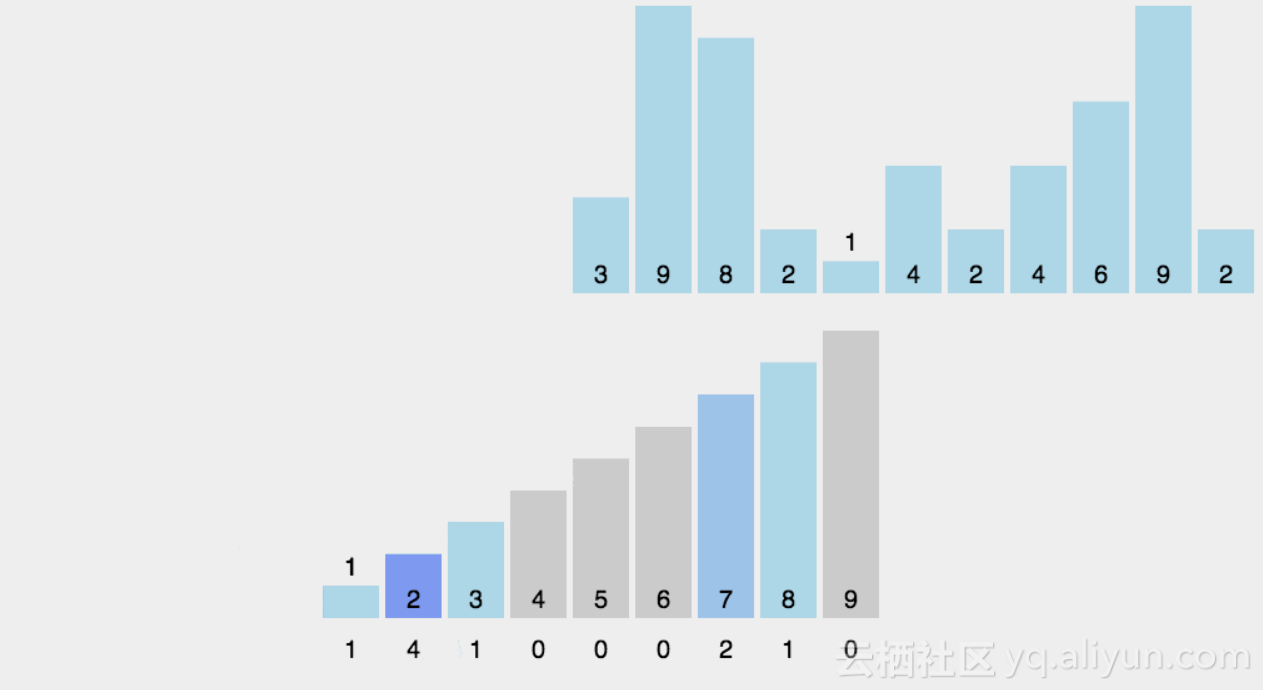2. Java 代码实现

public class CountingSort implements IArraySort {

@Override
public int[] sort(int[] sourceArray) throws Exception {
// 对 arr 进行拷贝，不改变参数内容
int[] arr = Arrays.copyOf(sourceArray, sourceArray.length);

int maxValue = getMaxValue(arr);

return countingSort(arr, maxValue);
}

private int[] countingSort(int[] arr, int maxValue) {
int bucketLen = maxValue + 1;
int[] bucket = new int[bucketLen];

for (int value : arr) {
bucket[value]++;
}

int sortedIndex = 0;
for (int j = 0; j < bucketLen; j++) {
while (bucket[j] > 0) {
arr[sortedIndex++] = j;
bucket[j]--;
}
}
return arr;
}

private int getMaxValue(int[] arr) {
int maxValue = arr;
for (int value : arr) {
if (maxValue < value) {
maxValue = value;
}
}
return maxValue;
}

}

●  在额外空间充足的情况下，尽量增大桶的数量
●  使用的映射函数能够将输入的 N 个数据均匀的分配到 K 个桶中

1. 什么时候最快

2. 什么时候最慢

3. Java 代码实现

public class BucketSort implements IArraySort {

private static final InsertSort insertSort = new InsertSort();

@Override
public int[] sort(int[] sourceArray) throws Exception {
// 对 arr 进行拷贝，不改变参数内容
int[] arr = Arrays.copyOf(sourceArray, sourceArray.length);

return bucketSort(arr, 5);
}

private int[] bucketSort(int[] arr, int bucketSize) throws Exception {
if (arr.length == 0) {
return arr;
}

int minValue = arr;
int maxValue = arr;
for (int value : arr) {
if (value < minValue) {
minValue = value;
} else if (value > maxValue) {
maxValue = value;
}
}

int bucketCount = (int) Math.floor((maxValue - minValue) / bucketSize) + 1;
int[][] buckets = new int[bucketCount];

// 利用映射函数将数据分配到各个桶中
for (int i = 0; i < arr.length; i++) {
int index = (int) Math.floor((arr[i] - minValue) / bucketSize);
buckets[index] = arrAppend(buckets[index], arr[i]);
}

int arrIndex = 0;
for (int[] bucket : buckets) {
if (bucket.length <= 0) {
continue;
}
// 对每个桶进行排序，这里使用了插入排序
bucket = insertSort.sort(bucket);
for (int value : bucket) {
arr[arrIndex++] = value;
}
}

return arr;
}

/**
* 自动扩容，并保存数据
*
* @param arr
* @param value
*/
private int[] arrAppend(int[] arr, int value) {
arr = Arrays.copyOf(arr, arr.length + 1);
arr[arr.length - 1] = value;
return arr;
}

}

1. 基数排序 vs 计数排序 vs 桶排序

●  基数排序：根据键值的每位数字来分配桶；
●  计数排序：每个桶只存储单一键值；
●  桶排序：每个桶存储一定范围的数值；

2. LSD 基数排序动图演示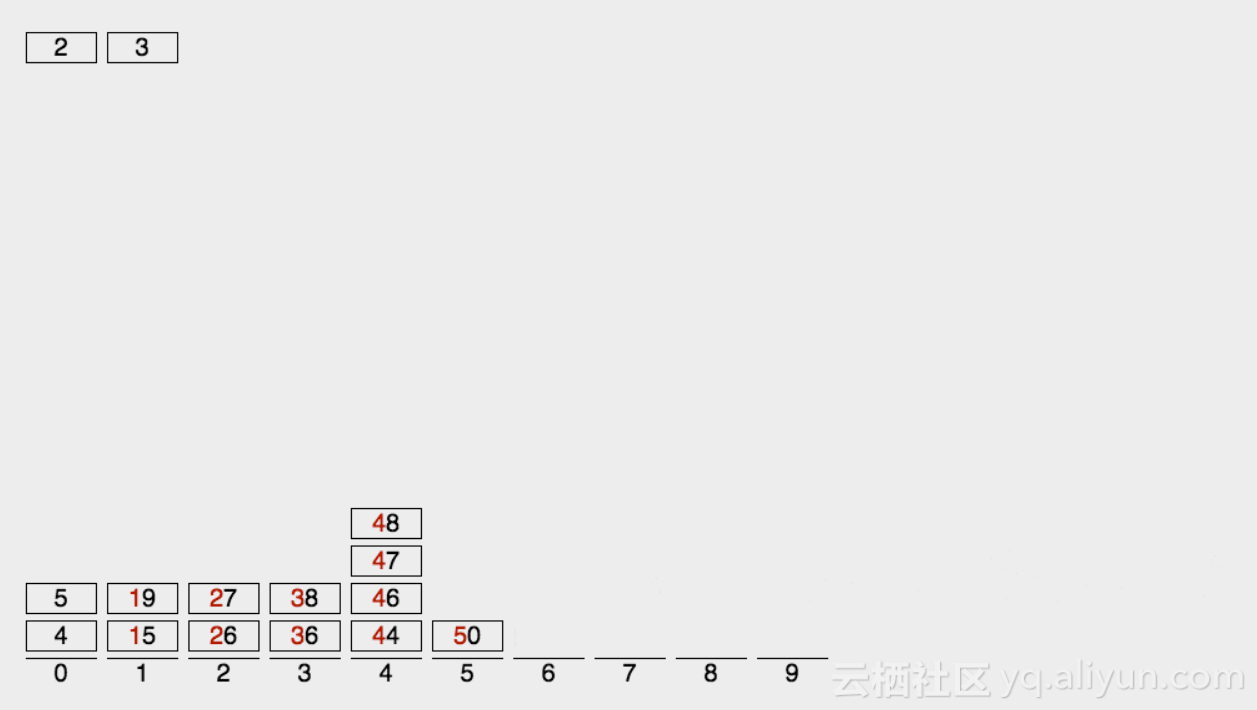3. Java 代码实现

/**
* 基数排序
* 考虑负数的情况还可以参考： https://code.i-harness.com/zh-CN/q/e98fa9
*/
public class RadixSort implements IArraySort {

@Override
public int[] sort(int[] sourceArray) throws Exception {
// 对 arr 进行拷贝，不改变参数内容
int[] arr = Arrays.copyOf(sourceArray, sourceArray.length);

int maxDigit = getMaxDigit(arr);
}

/**
* 获取最高位数
*/
private int getMaxDigit(int[] arr) {
int maxValue = getMaxValue(arr);
return getNumLenght(maxValue);
}

private int getMaxValue(int[] arr) {
int maxValue = arr;
for (int value : arr) {
if (maxValue < value) {
maxValue = value;
}
}
return maxValue;
}

protected int getNumLenght(long num) {
if (num == 0) {
return 1;
}
int lenght = 0;
for (long temp = num; temp != 0; temp /= 10) {
lenght++;
}
return lenght;
}

private int[] radixSort(int[] arr, int maxDigit) {
int mod = 10;
int dev = 1;

for (int i = 0; i < maxDigit; i++, dev *= 10, mod *= 10) {
// 考虑负数的情况，这里扩展一倍队列数，其中 [0-9]对应负数，[10-19]对应正数 (bucket + 10)
int[][] counter = new int[mod * 2];

for (int j = 0; j < arr.length; j++) {
int bucket = ((arr[j] % mod) / dev) + mod;
counter[bucket] = arrayAppend(counter[bucket], arr[j]);
}

int pos = 0;
for (int[] bucket : counter) {
for (int value : bucket) {
arr[pos++] = value;
}
}
}

return arr;
}

/**
* 自动扩容，并保存数据
*
* @param arr
* @param value
*/
private int[] arrayAppend(int[] arr, int value) {
arr = Arrays.copyOf(arr, arr.length + 1);
arr[arr.length - 1] = value;
return arr;
}
}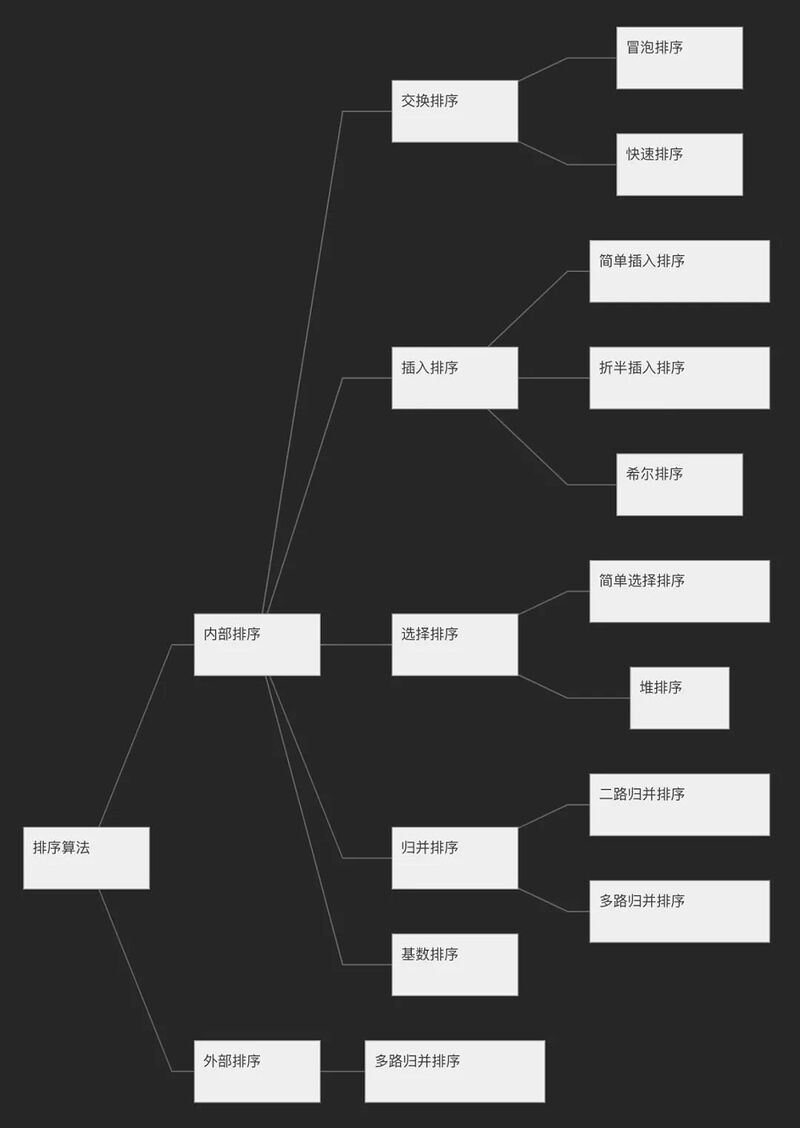42 0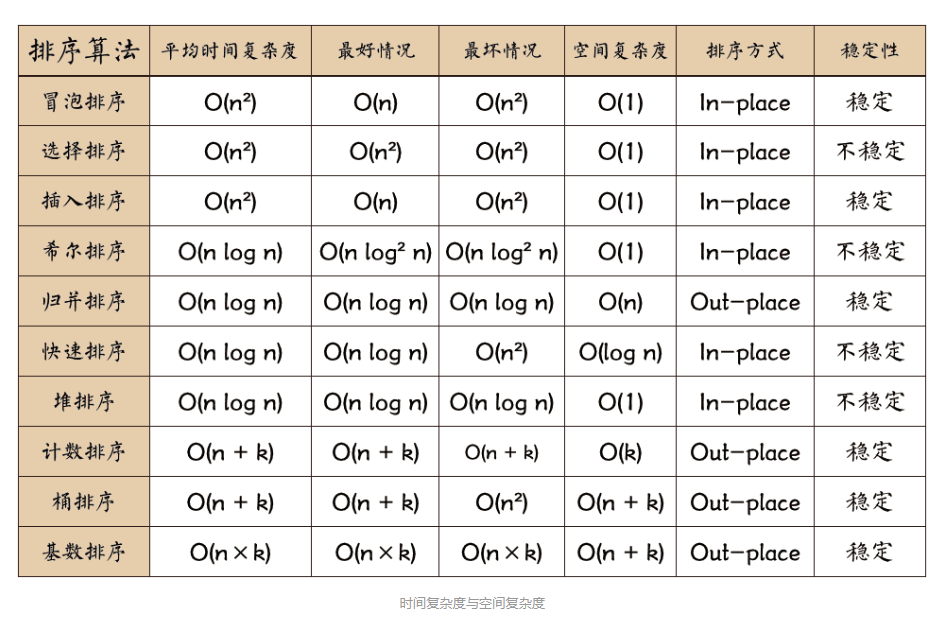1742 01109 011月14日云栖精选夜读 | 动画+原理+代码，解读十大经典排序算法

3199 01393 01046 01676 0778 0【读书笔记《Android游戏编程之从零开始》】18.游戏开发基础（碰撞检测）
1416 0

26个GitHub上好玩又有挑战的前端项目（涵盖初中高阶）4761

【科技少年】Python绘画编程第一课272020

21个GitHub上好用又有趣的移动端项目（涵盖初中高阶）2681

10个GitHub上适合练手的后端项目（涵盖初中高阶）10471

【新人赛】工业蒸汽量预测建模算法代码开源分享合集113641199

《优酷响应式布局技术全解析》

H5动画在移动平台上的性能优化实践